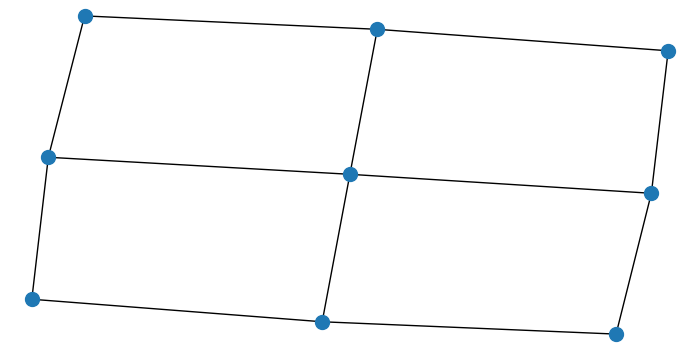# Matplotlib â€“ Drawing lattices and graphs with Networkx

To draw lattices and graphs with networkx, we can take the following steps −

• Import networkx and pyplot.
• Set the figure size and adjust the padding between and around the subplots.
• Use nx.grid_2d_graph(3, 3) to get a two-dimensional grid graph. The grid graph has each node connected to its four nearest neighbors.
• Draw the graph G with Matplotlib.
• To display the figure, use show() method.

## Example

# Import networkx and pyplot
import networkx as nx
from matplotlib import pyplot as plt

# Set the figure size
plt.rcParams["figure.figsize"] = [7.00, 3.50]
plt.rcParams["figure.autolayout"] = True

# Draw the graph
G = nx.grid_2d_graph(3, 3)
nx.draw(G, node_size=100)

plt.show()

## Output

It will produce the following output